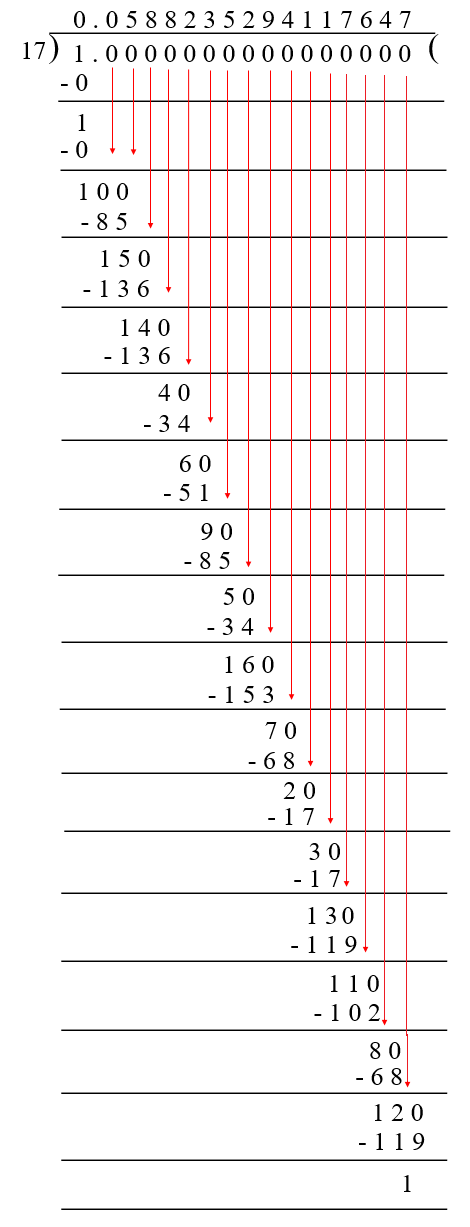# vs.eyeandcontacts.com

## Chapter 1 Number Systems Exercise 1.3

Question 1: Write the following in decimal from and say what kind of decimal expansion each has:
i) 36/100
ii) 1/11
iii) 4 1/8
iv) 3/13
v) 2/11
vi) 329/400

i) 36/100 = 0.36 (Terminating because denominator contains the factors of powers of 2 and 5)
ii) 1/11  = 0.09 (Non-Terminating Repeating because the denominator does not contain the factors of powers of 2 and 5)
iii) 4 1/8 = 4.125 (Terminating because denominator contains the factors of powers of 2)
iv) 3/13 = 0.230… (Non-Terminating Repeating because denominator does not contain the factors of powers of 2 and 5)
v) 2/11 = 0.18 (Non-Terminating Repeating because denominator does not contain the factors of powers of 2 and 5)
vi) 329/400 = 0.8225 (Terminating because denominator contains the factors of powers of 2 and 5)

Question 2: We know that 1/7 = 0.142857. Can you predict what the decimal expansion of 2/7, 3/7, 4/7, 5/7, 6/7 are, without actually doing the long division? If so, how?
[Hint: Study the remainders while finding the value of 1/7 carefully]

Since, 1/7 = 0.142857
2 × 1/7 = 2/7
2 × 0.142857 = 0.285714
3 × 1/7 = 3/7
3 × 0.142857 = 0.428571
4 × 1/7 = 4/7
4 × 0.142857 = 0.571428
5 × 1/7 = 5/7
5 × 0.142857 = 0.714285
6 × 1/7 = 6/7
6 × 0.142857 = 0.857142

Question 3: Express the following in the form of p/q, where p and q are integers and q ≠ 0.
i) 0.6
ii) 0.47
iii) 0.001

i)
x = 0.666…
10x = 6.666…
x = 0.666…
9x = 6
x = 6/9
x = 2/3

ii)
x = 0.4777…
10x = 4.777…
100x = 47.777…
-10x = 4.777….
90x = 43
x = 43/90

iii)
x = 0.001001001…
1000x = 1.001001001…
-x = 0.001001001…
999x = 1
x = 1/999

Question 4: Express 0.99999… in the form p/q. Are you surprised by your answer? With your teacher and classmates discuss why the answer makes sense.

x = 0.999…
10x = 9.999…
-x = 0.999…
9x = 9
x = 9/9 or 1

Question 5: What can the maximum number of digits be in the repeating block of digits in the decimal expansion of 1/17? Perform the division to check your answer.Question 6: Look at several examples of rational numbers in the form p/q (q ≠ 0), where p and q are integers with no common factors other than 1 and having terminating decimal representations (expansions). Can you guess what property q must satisfy?
The prime factorisation of q has only powers of 2 or powers of 5 or both.

Question 7: Write three numbers whose decimal expansions are non-terminating non-recurring.

0.956509267…
0.7200710201…
0.76700769907…

Question 8: Find three different irrational numbers between the rational numbers 5/7 and 9/11.

5/7 × 11/11 = 55/77
9/11 × 7/7 = 63/77
55/77 = 0.7142199…
63/77 = 0.818181…
Hence, the rational numbers between 5/7 and 9/11 are
0.724130…
0.796120…
0.80010001…

Question 9: Classify the following numbers as rational or irrational according to their type:
i) √23
ii) √225
iii) 0.3796
iv) 7.478478…
v) 1.101001000100001…

i) √23 = 4.795…
As the number is Non-Terminating Non-Recurring, it is an irrational number.
ii) √225 = 15
As the number can be represent in the p/q form, it is an rational number.
iii) 0.3796
As the number is terminating, it is an rational number.
iv) 7.478478…
As the number is Non-Terminating Non-Recurring, it is an irrational number.
v) 1.101001000100001…
As the number is Non-Terminating Non-Recurring, it is an irrational number.